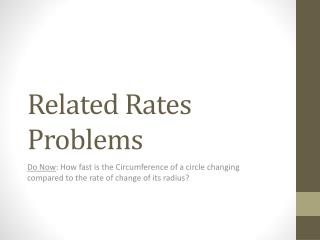DownloadDownload PresentationRelated Rates Problems

Related Rates Problems

Download PresentationRelated Rates Problems

- - - - - - - - - - - - - - - - - - - - - - - - - - - E N D - - - - - - - - - - - - - - - - - - - - - - - - - - -
Presentation Transcript

1. Related Rates Problems Do Now: How fast is the Circumference of a circle changing compared to the rate of change of its radius?

2. Problem #1 • How fast is the area of a square increasing at the instant when the sides are 4 feet long if the sides increase at a rate of 1.5 ft/min?

3. Solution #1 • (units?)

4. Problem #2 • Suppose . At the instant when x=1 and y=3, x is decreasing at 2 units/sec and y is increasing at 2 units/sec. How fast is z changing at this instant? Increasing or decreasing?

5. Solution #2 • units? • Units/sec

6. Problem #3 • Let be the acute angle of a right triangle with legs of length x and y. At a certain instant, x=4 and is increasing at 2 units/second, while y=3 and is decreasing at ½ units/sec. How fast is changing at that instant? Is it increasing or decreasing?

7. Solution #3

8. Problem #4 • Assume that oil spilled from a ruptured tanker spreads in a circular pattern whose radius increases at a constant rate of 2 ft/sec. How fast is the area of the spill increasing when the radius of the spill is 60 ft?

9. Solution #4

10. Problem #5 • Suppose that a spherical balloon is inflated at the rate of 10 cubic centimeters per minute. How fast is the radius of the balloon increasing when the radius is 5 centimeters?

11. Solution #5

12. Problem #6 • One end of a 13-foot ladder is on the floor and the other rests on a vertical wall. If the bottom end is drawn away from the wall at 3 feet per second, how fast is the top of the ladder sliding down the wall when the bottom of the ladder is 5 feet from the wall?

13. Solution #6 • (Where did I get 12?)

14. Problem #7 • One end of a 13-foot ladder is on the floor and the other rests on a vertical wall. If the bottom end is drawn away from the wall at 3 feet per second, how fast is the angle of elevation of the ladder changing when the bottom of the ladder is 5 feet from the wall?

15. Solution #7

16. Problem #8 • A baseball diamond is a square whose sides are 90 ft long. Suppose that a player running from second base to third base has a speed of 30 ft/sec at the instant when he is 20 feet from third base. At what rate is the player’s distance from home plate changing at that instant?

17. Solution #8 • At this instant

18. Problem #9 • A rocket is rising vertically at 880 ft/sec. At the instant when the rocket is 4000 feet high, how fast must a camera’s elevation angle change to keep the rocket in sight if the camera is 3000 ft away from the launching pad?

19. Solution #9 • . • At this moment, the hypotenuse is .